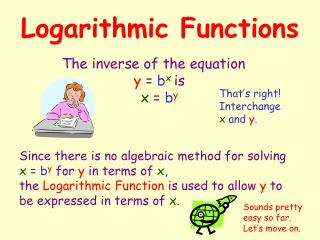DownloadDownload PresentationLogarithmic Functions

# Logarithmic Functions

Télécharger la présentation## Logarithmic Functions

- - - - - - - - - - - - - - - - - - - - - - - - - - - E N D - - - - - - - - - - - - - - - - - - - - - - - - - - -
##### Presentation Transcript

1. Logarithmic Functions The inverse of the equation y = bx is x = by That’s right! Interchange x and y. Since there is no algebraic method for solving x = by for y in terms of x, the Logarithmic Function is used to allow y to be expressed in terms of x. Sounds pretty easy so far. Let’s move on.

2. Let’s Take a Closer Look at Some Logs A logarithm is really an exponent written in a different form. Now let’s bring in the logs. The equation y = bx is an exponential function Written in logarithmic form, the equation y = bx would be x = logba Let’s break this down. b is the base x is the exponent y is the value of bx We read this as xis the logarithm of awith base b

3. Breaking Down Logs Let’s look at a log piece by piece. The equation x = logba is a logarithmic function Hey! I’ve seen this before. Let’s break this down. It’s Sam Ting as breaking down exponential functions. b is the base x is the exponent a is the value of bx That was easy

4. Comparing Logarithmic form and exponential form Exponential Form Logarithmic Form y = bx x= logb a 32 = 25 5= log232 3= log8512 512 = 83 Asi De Facil 81 = 34 4= log381 125 = 53 3= log5125

5. Logarithms with Variables In each equation, find the value of the variable x= log6 36 3= logb 125 3= log4a 43=a 6x= 36 b3= 125 since 43 =64, a = 64 since 62 = 36, x = 2 since 53 = 125, b = 5 This looks a little harder. Maybe I should use a real calculator for this one. Hey, I can just use my calculator for this. That was easy

6. More Logarithms with Variables In each equation, find the value of the variable 5= log8 a x= log7 2,401 3= logb 6,859 85 =a 7x = 2,401 b3 = 6,859 since 85 =32,768, a = 32,768 since 74 = 2,401, x = 4 since 193 = 6,859, b = 19 Hey, those are some pretty big numbers. I hope my calculator knows how to do this. That was easy

7. 10 10 Common Logs Any logarithm with base 10 is a Common Log When writing a common logarithm, the base is usually omitted. So,5= log10 100,000 and5= log100,000 are Sam Ting. Let’s compare Logarithmic Form and Exponential Form of some Common Logs. Logarithmic Form Exponential Form That was easy 3= log1,000 1,000 = 103 6= log1,000,000 1,000,000 = 106 4= log10,000 10,000 = 104

8. Common Logs with Variables In each equation, find the value of the variable 7= loga x= log100 107 =a 10x =100 since 107 =10,000,000, then a = 10,000,000 since 102 =100, then x = 2 write the proper number of zeros count the zeros That was easy Hey, I don’t even need a calculator for this!

9. More Common Logs with Variables Find the value of the variable to the nearest one hundredth 2.865= loga x= log1,345 102.865 =a 10x =1,345 What’s the proper number of zeros? Hey, there’s no zeros to count. We could use the 10x key on our calculator. We could use the LOG key on our calculator. 102.865 =732.82 LOG (1,345) = 3.13 That was easy

10. Change of Base How can I get my calculator to evaluate logs in bases other than base 10? That’s easy, just use the Change of Base Formula It’s time to push the easy button once again! x= log8512 = 3 x= log12248,832 = 5

11. More Change of Base Let’s throw some decimals into the mix. That was easy x= log4.591.125 = 3 This stuff is too easy. Soon I’ll have to buy new batteries for my easy button. x= log432 = 2.5 x= log8.1251,986.597 = 3.625